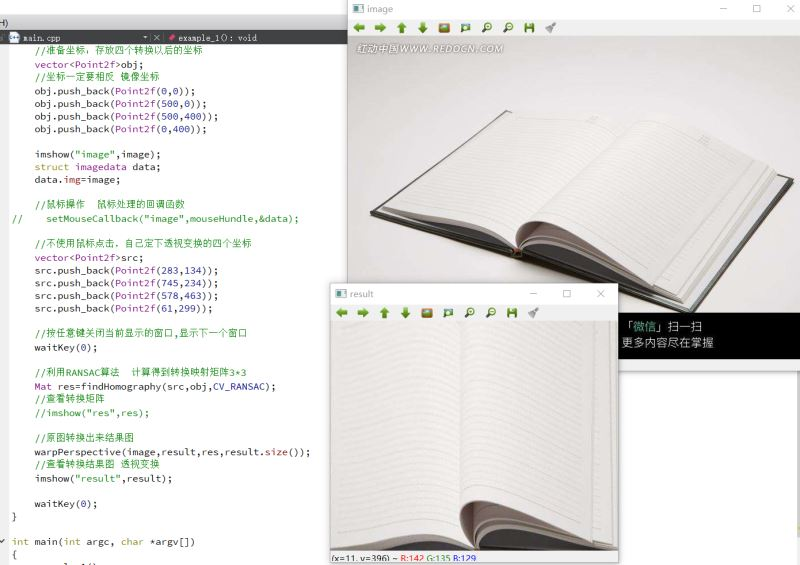﻿ OpenCV透视变换应用之书本视图矫正+广告屏幕切换_C 语言_脚本之家
C 语言# OpenCV透视变换应用之书本视图矫正+广告屏幕切换

## 一、透视变换的实际应用场景## 二、案例了解透视变换--书本视图矫正```#include <iostream>
#include <opencv2/opencv.hpp>
#include<vector>
using namespace cv;
using namespace std;

//鼠标操作 自己准备结构体
struct imagedata
{
Mat img;//目标图像 用于点击 确定坐标
vector<Point2f> points;//存放原图的坐标 通过鼠标的点击进行存放
};

//鼠标操作的回调函数:用于选择四个角的点（使用方法：从左上角开始顺时针选择四个点，选完之后回车操作）
void mouseHundle(int event,int x,int y,int flag,void *arg)
{
//强制转换
struct imagedata * d = (struct imagedata*)arg;
//如果按下的是鼠标左键
if(event==EVENT_LBUTTONDOWN)
{
//用圆形来标记下鼠标按下左键标记的位置
circle(d->img,Point(x,y),3,Scalar(255,0,0),3,CV_AA);//在图上标记,圆心为点击的位置
imshow("image",d->img);//原窗口上进行显示标记点
//透视变换 需要使用四个点的坐标
if(d->points.size()<4)
{
d->points.push_back(Point2f(x,y));//把点击下来的坐标进行存储
}
}
}

void example_1()
{
Mat result=Mat::zeros(400,500,CV_8UC1);//最终结果显示 单通道

//准备坐标：存放四个转换以后的坐标
vector<Point2f>obj;
//坐标一定要相反 镜像坐标
obj.push_back(Point2f(0,0));
obj.push_back(Point2f(500,0));
obj.push_back(Point2f(500,400));
obj.push_back(Point2f(0,400));

imshow("image",image);
struct imagedata data;
data.img=image;

//鼠标操作  鼠标处理的回调函数
setMouseCallback("image",mouseHundle,&data);

//按任意键关闭当前显示的窗口,显示下一个窗口
waitKey(0);

//利用RANSAC算法  计算得到转换映射矩阵3*3
Mat res=findHomography(data.points,obj,CV_RANSAC);
//查看转换矩阵
//imshow("res",res);

//原图转换出来结果图
warpPerspective(image,result,res,result.size());
//查看转换结果图 透视变换
imshow("result",result);

waitKey(0);
}

int main(int argc, char *argv[])
{
example_1();
return 0;
}``````    //鼠标操作  鼠标处理的回调函数
//setMouseCallback("image",mouseHundle,&data);

//不使用鼠标点击，自己定下透视变换的四个坐标
vector<Point2f>src;
src.push_back(Point2f(283,134));
src.push_back(Point2f(745,234));
src.push_back(Point2f(578,463));
src.push_back(Point2f(61,299));

//按任意键关闭当前显示的窗口,显示下一个窗口
waitKey(0);

//利用RANSAC算法  计算得到转换映射矩阵3*3
Mat res=findHomography(src,obj,CV_RANSAC);
//查看转换矩阵
//imshow("res",res);```## 三、案例了解透视变换--广告屏幕切换```#include <iostream>
#include <opencv2/opencv.hpp>
using namespace cv;
using namespace std;

struct imagedata
{
Mat img;//目标图像
vector <Point2f> points;//3D点
};

//鼠标操作函数:用于选择四个角的点（使用方法有顺序的，从左上角顺时针选择，选完之后回车）
void mouseHundle(int event,int x,int y,int flag,void *ptr)
{
struct imagedata * d=(struct imagedata*)ptr;
if(event==EVENT_LBUTTONDOWN)
{
//确定按下的是鼠标左键
//用圆形标记一下鼠标按下左键标记的位置
circle(d->img,Point(x,y),3,Scalar(255,0,0),3,CV_AA);//在图上标记,圆心为点击的位置

imshow("dst",d->img);//在dst背景图中显示鼠标选取的图片

if(d->points.size()<4)//只存下来，最先点的前四个点
{
d->points.push_back(Point2f(x,y));//把鼠标操作点击的点存起来
}
}
}

void example_2()
{

Mat dst=image2.clone();//背景图片 克隆

//存放原图坐标 想要切换的广告屏图片
vector <Point2f>obj;
obj.push_back(Point2f(0,0));
obj.push_back(Point2f(image1.cols,0));
obj.push_back(Point2f(image1.cols,image1.rows));
obj.push_back(Point2f(0,image1.rows));

//纽约时代广场图背景图显示
imshow("dst",dst);
struct imagedata data;
data.img =dst;

//鼠标操作 鼠标处理的回调函数
setMouseCallback("dst",mouseHundle,&data);
//按任意键关闭当前显示的窗口,显示下一个窗口
waitKey(0);

//计算得到转换映射矩阵 3*3  原图坐标转换为鼠标选择
Mat res = findHomography(obj,data.points,CV_RANSAC);
//imshow("res",res);
//透视变换
warpPerspective(image1,dst,res,dst.size());
//仅有的却换后的广告图图片
//imshow("image1",dst);

Point pts;
for(int i=0;i<4;i++)
{
pts[i]=data.points[i];
}
//鼠标点选的四个坐标的区域填充成黑色
fillConvexPoly(image2,pts,4,Scalar(0),CV_AA);
//imshow("image2",image2);

//将仅有的却换后的广告图图片 和  鼠标点选的四个坐标的区域填充成黑色的背景图图片 叠加
image2+=dst;
//显示出最后广告换屏结果图片
imshow("final",image2);

waitKey(0);
}

int main(int argc, char *argv[])
{
example_2();
return 0;
}
```